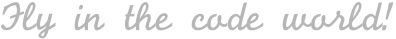#### 1. 复杂度分析（时间、空间复杂度）—— Ficow 陪你学算法和数据结构

| Swift , 算法&数据结构

• 前言
• 时间复杂度
• 空间复杂度
• 总结

## 前言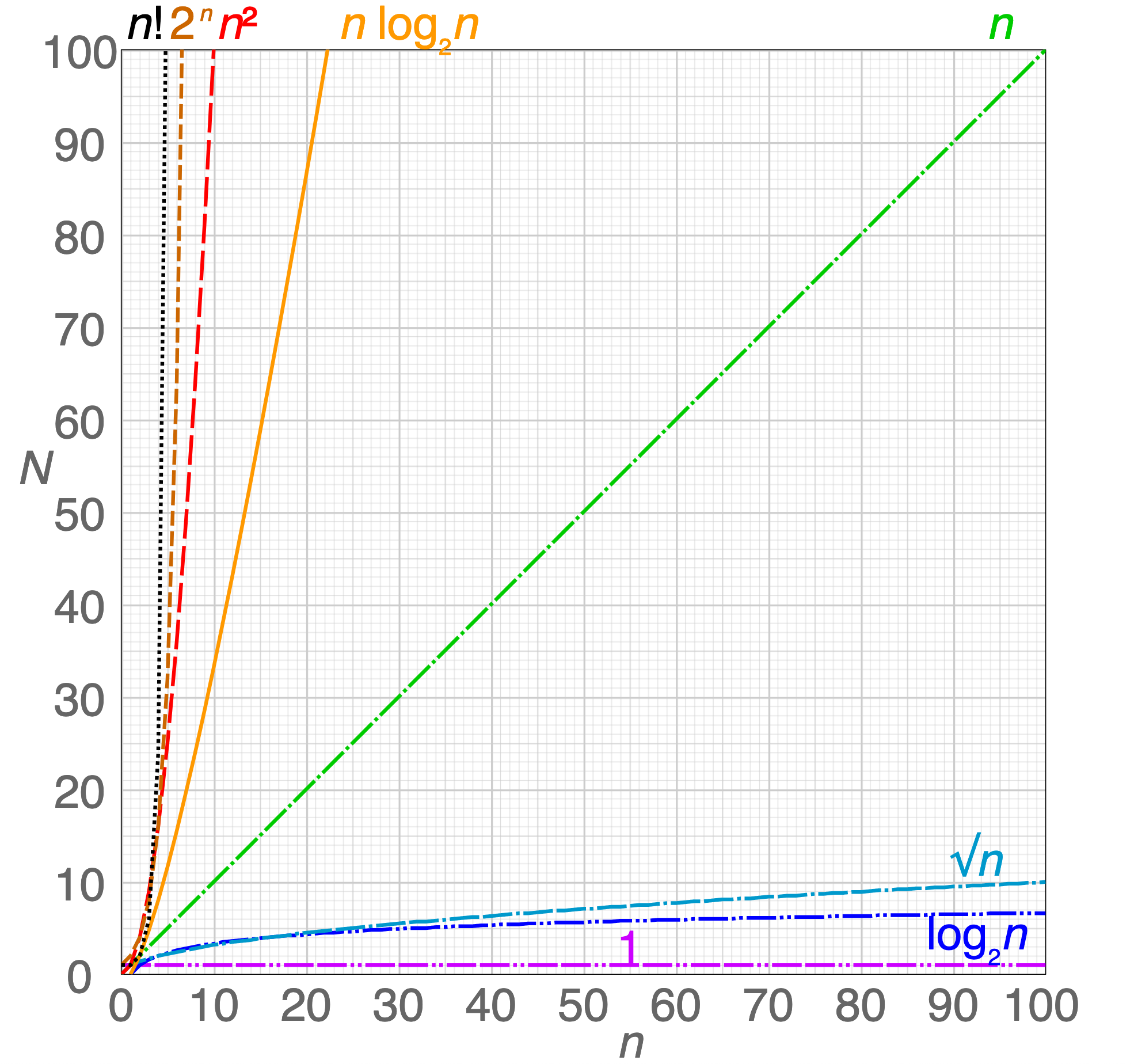• 复杂度分析
• 数组、链表、跳表
• 树、二叉树、二叉搜索树
• 递归（代码模板非常给力）

## 时间复杂度

##### 常见的时间复杂度函数：

O(1)、O(n)、O(nlogn) 、O(n²)、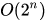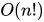• O(1) 时间复杂度：
``````let n = 1000
print(n)
``````
• O(n) 时间复杂度（一层循环）：

``````let n = 100
for i in 0..<n {
print(i)
}
for j in 0..<n {
print(j)
}
``````
• O(nlogn) 时间复杂度：

``````var n = 10000, i = 1
while i < n {
print(i)
i *= 2
}
``````
• O(n²) 时间复杂度（两层循环嵌套）：

``````for i in 0..<100 {
for j in 0..<1000 {
print(i*j)
}
}
``````
•时间复杂度：

``````func fib(_ n: Int) -> Int {
if n < 2 { return n }
return fib(n - 1) + fib(n - 2)
}
fib(50)
``````

• 暴力求解，循环累加，O(n) 时间复杂度：

``````let n = 10000
var sum = 0
for i in 0..<n {
sum += i
}
print(sum)
``````
• 采用数学公式进行计算，O(1) 时间复杂度：

``````let n = 10000
let sum = n * (n + 1) / 2
print(sum)
``````

• 和面试官确认题目的意思，保证理解无误（很多人由于思维模式或者情绪问题，无法准确地理解他人的实际需求）；
• 想出所有可能的解法，并比较它们的时间、空间复杂度（不要急着提交，三思而后行）；
• 找出运行时间最短、内存占用最少的最优解法；
• 写代码并测试结果；

#### 如何计算递归的时间复杂度？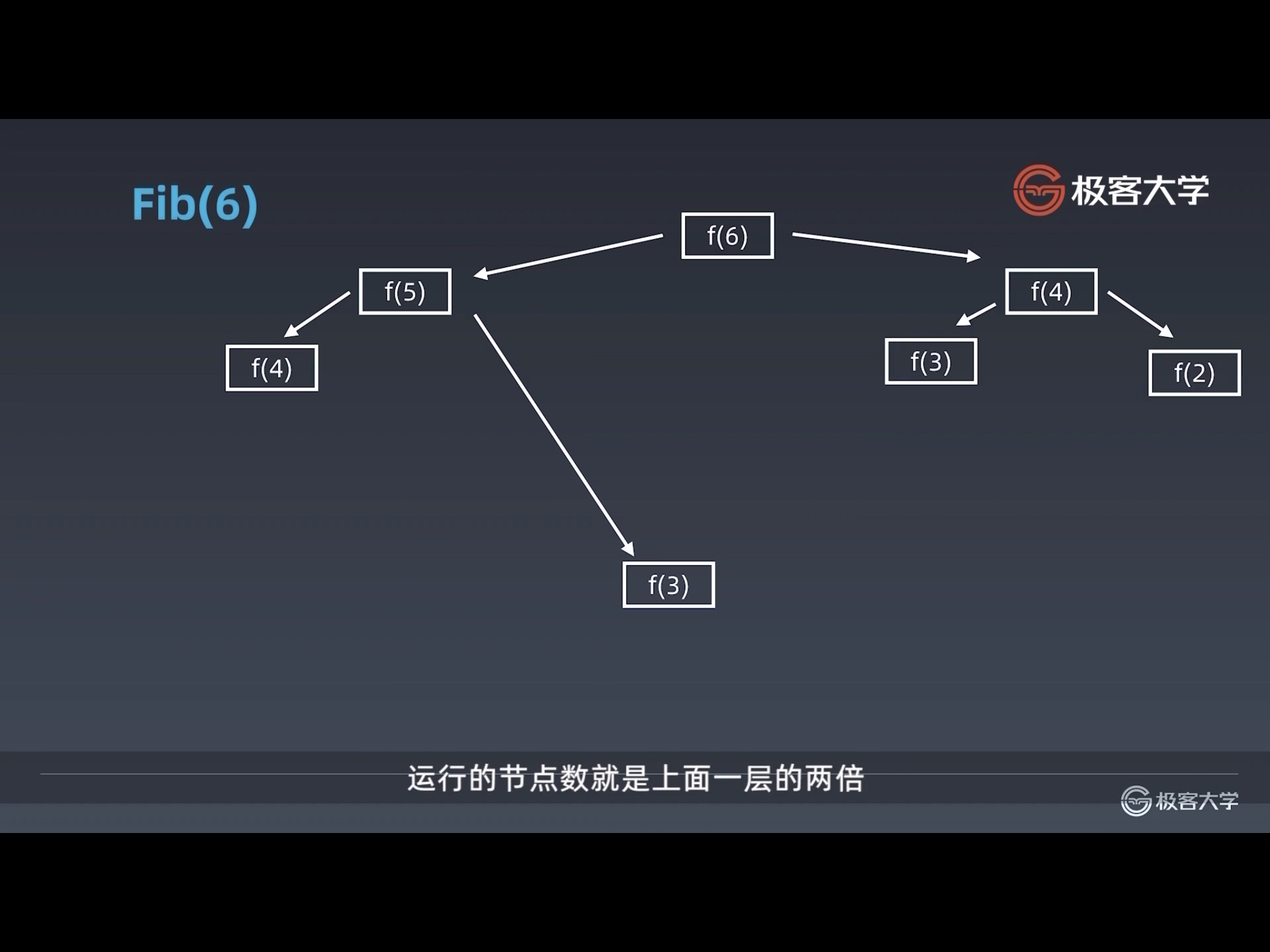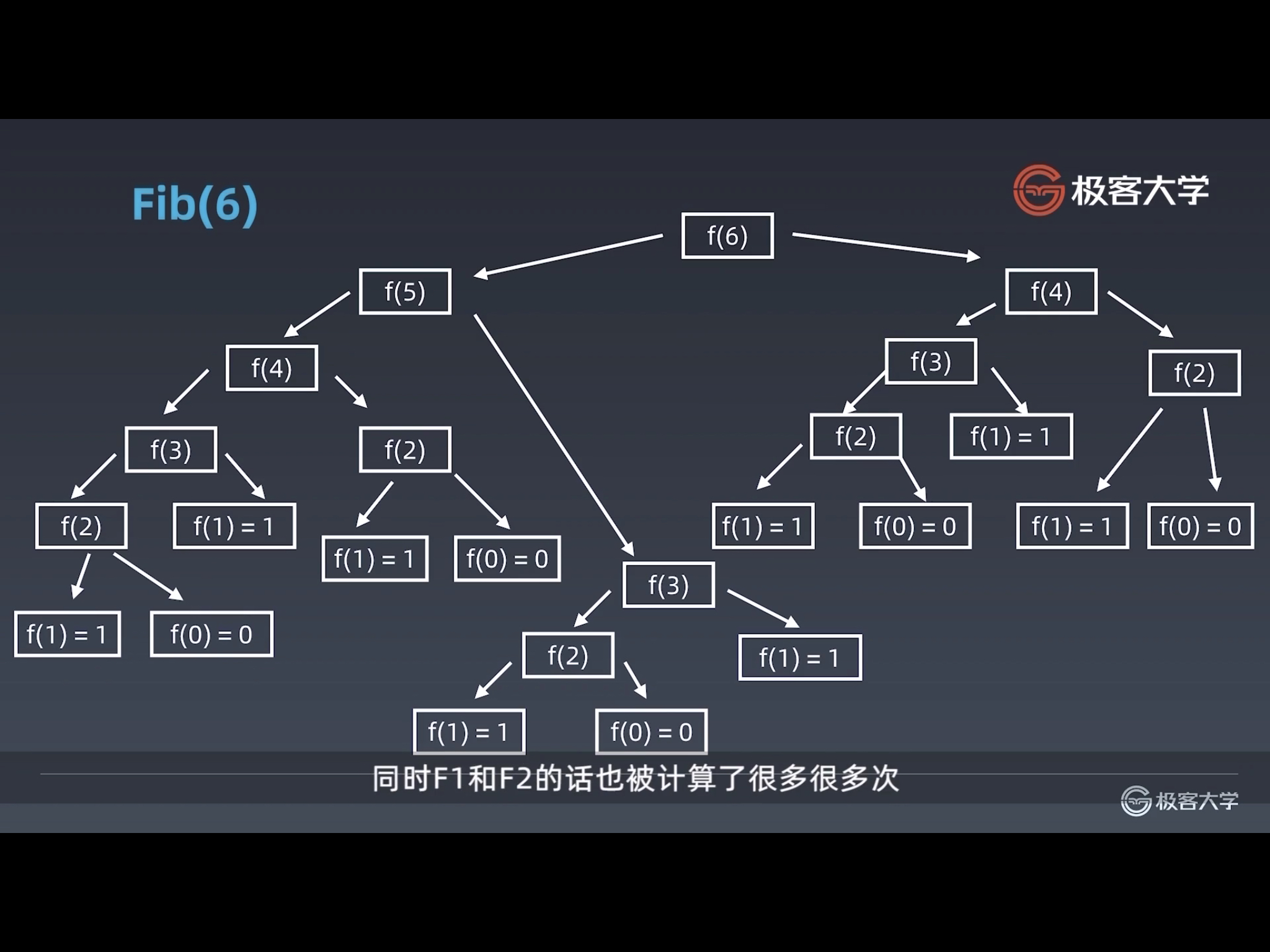• 缓存计算结果；
• 将递归调用改为循环；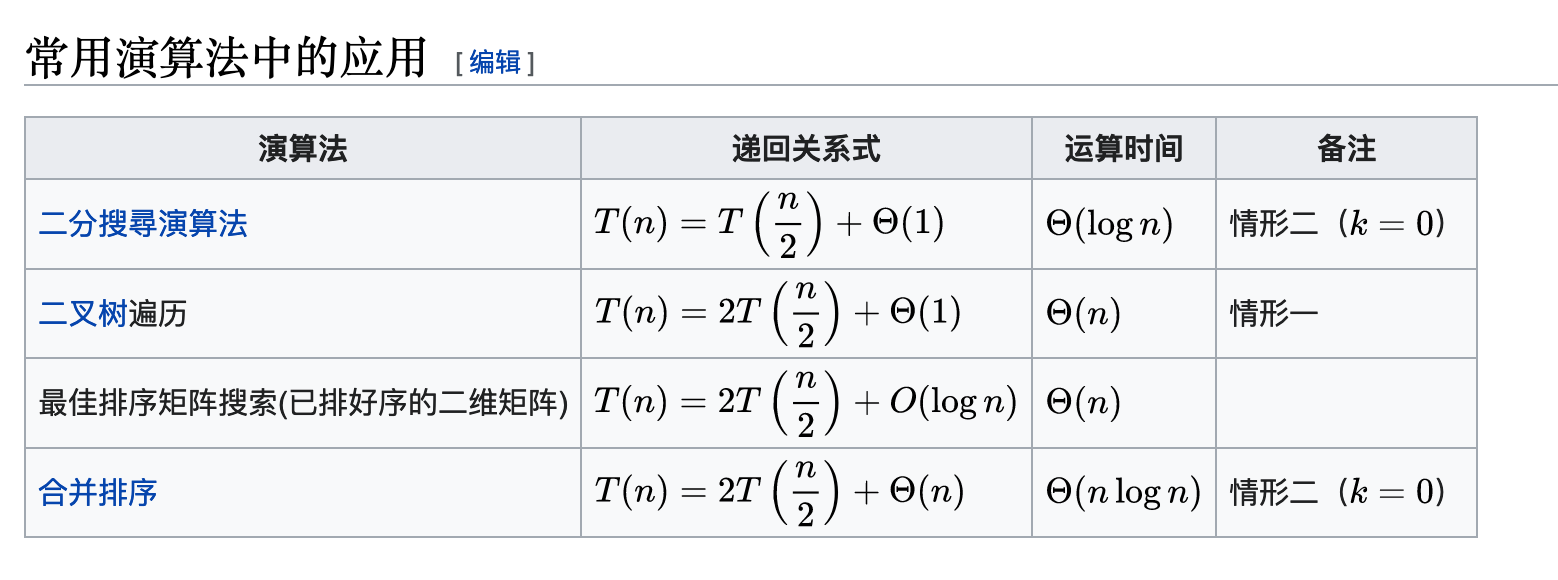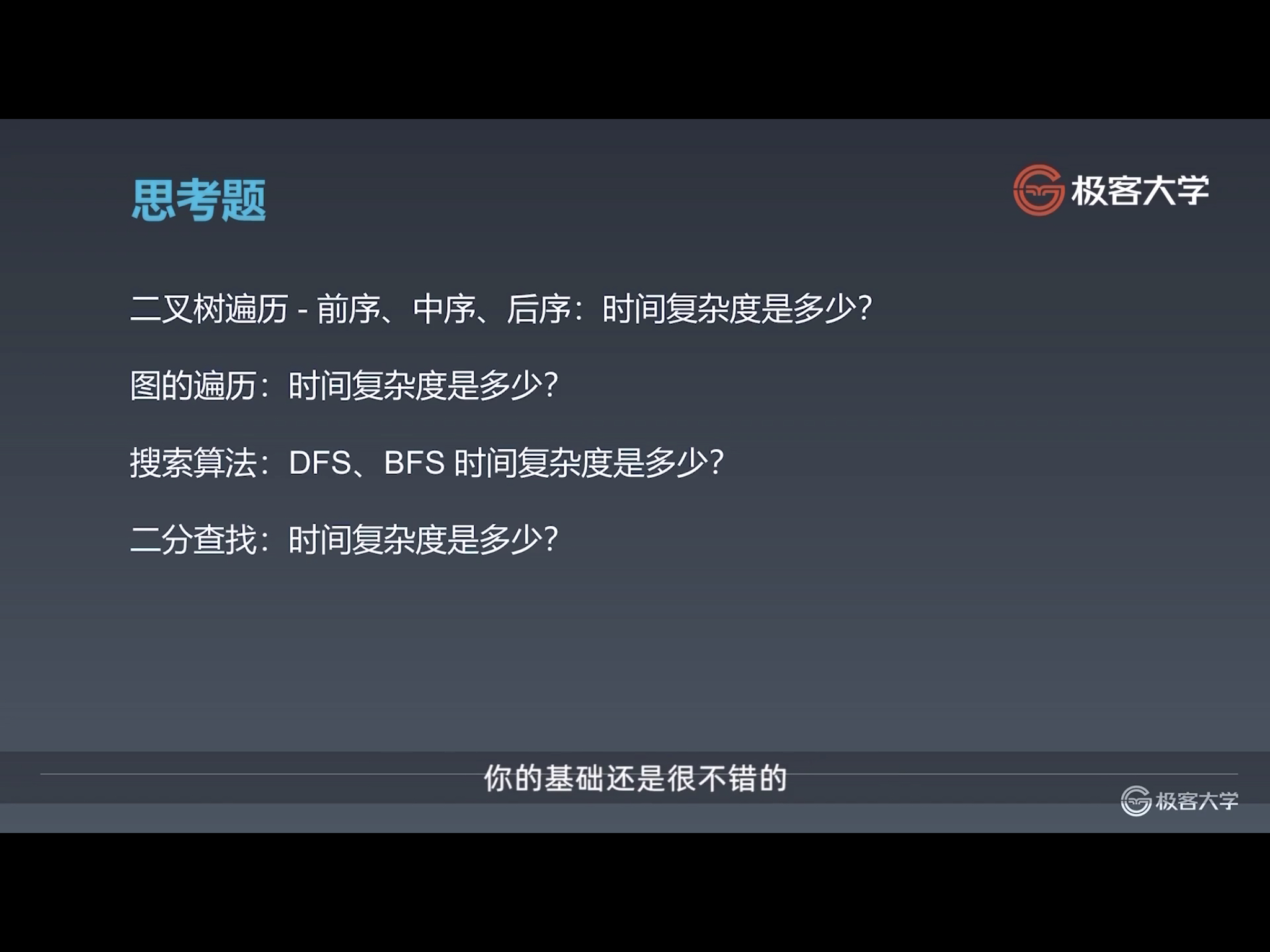## 空间复杂度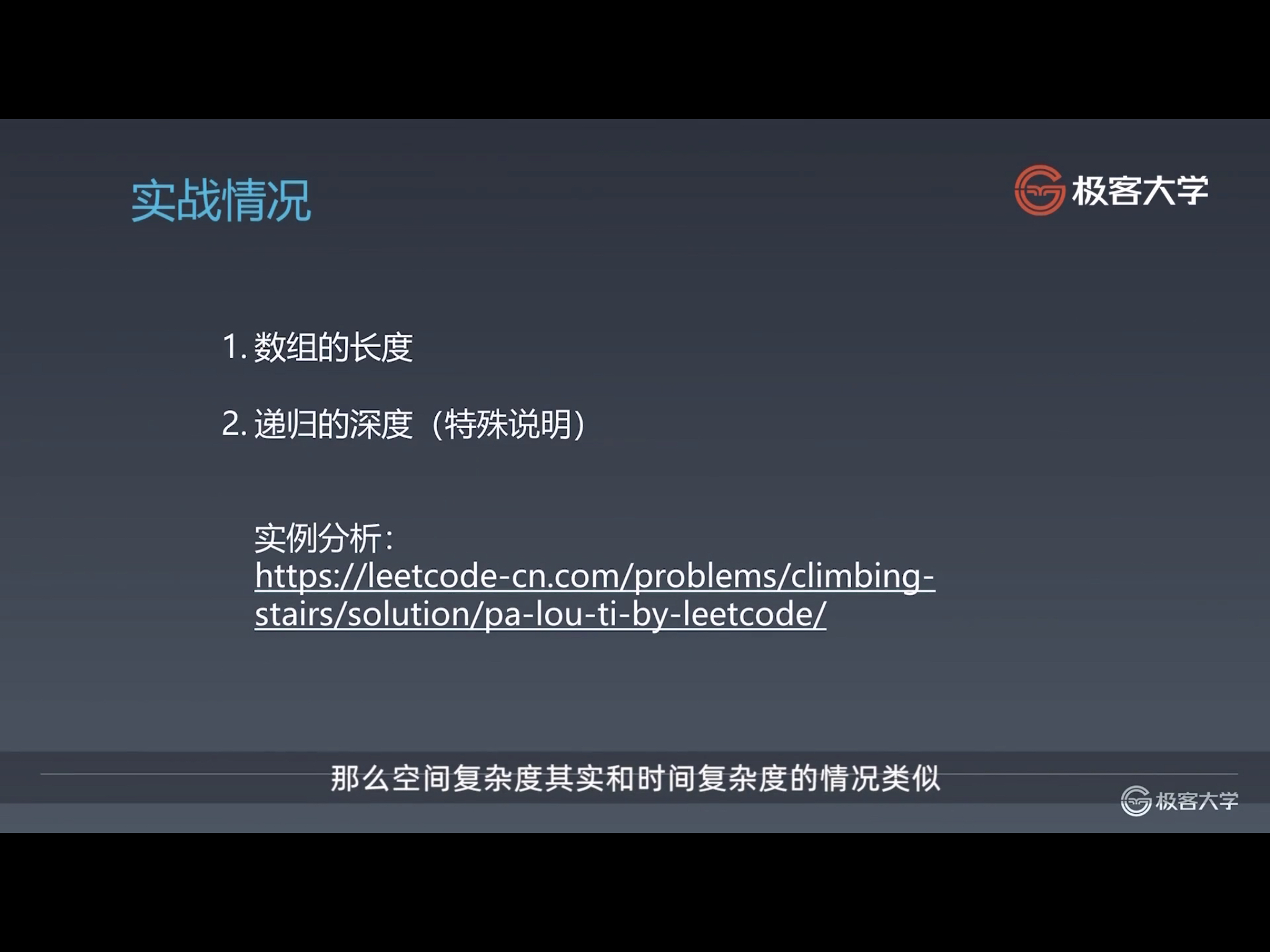##### 计算原则：
• 如果用了数组，数组所占用的内存空间就是其空间复杂度。比如：一维数组就是 O(n)，行和列一样多的二维数组就是 O(n^2)。
• 如果用了递归，递归需要用到函数调用栈，递归调用的最大深度就是调用栈的最大深度，栈所占用的内存空间就是其空间复杂度。
• 如果又用了数组，又用了递归，两者中空间复杂度最大的就是最终的空间复杂度。

• 常用工具配置
• 基本功和编程指法
• 常见的时间、空间复杂度

## 总结

#### 复杂度分析的要点：

• 只看最高复杂度的运算；
• 常见的复杂度函数： O(1)、O(n)、O(nlogn) 、O(n²)、• 记住 `运算次数 N``数据规模 n` 之间的关系，脑海中要有这样一张图：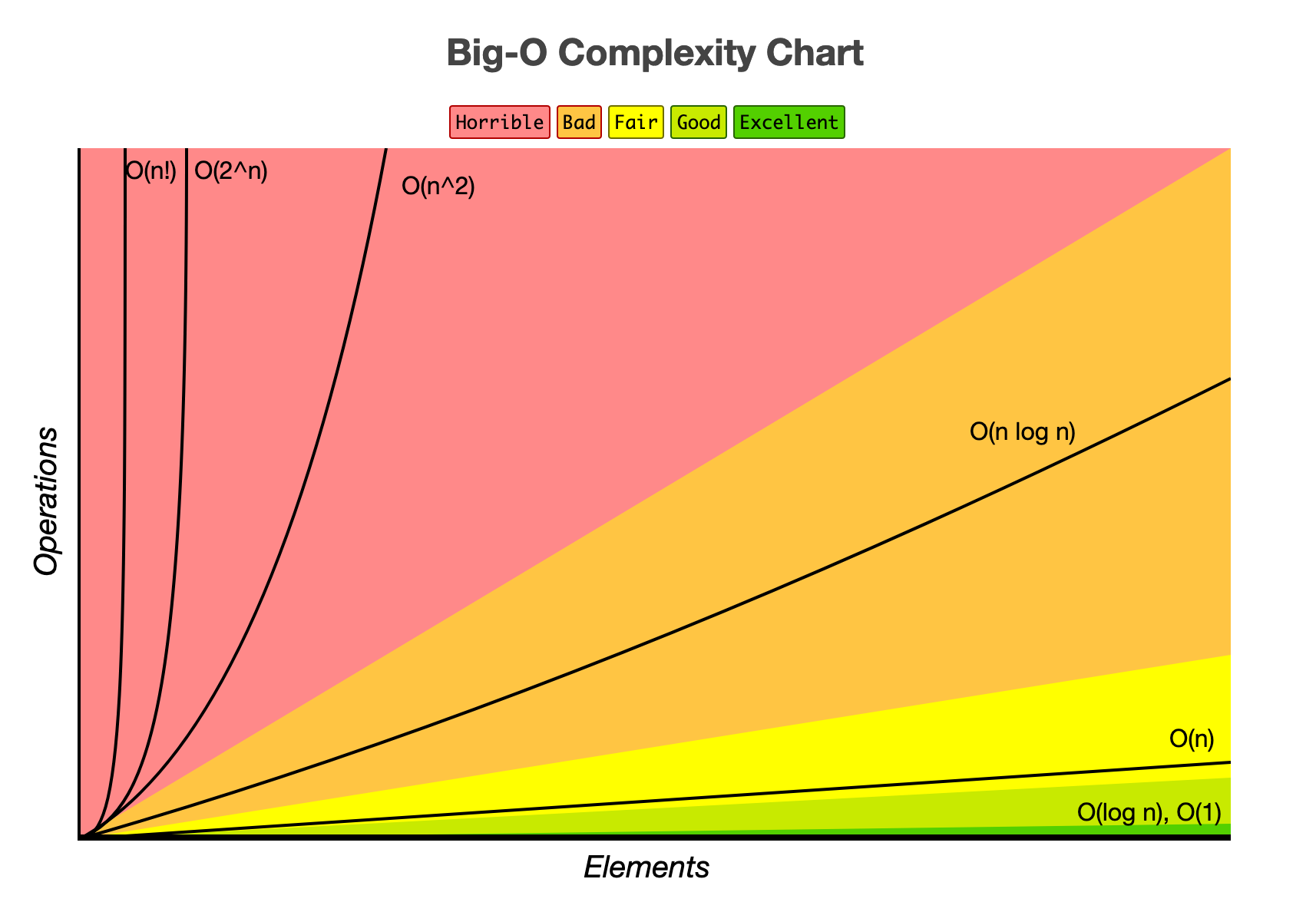#### 评论区(期待你的留言)